# FIRM VALUATION Firm Valuation Assumptions n Corporate taxes

• Slides: 19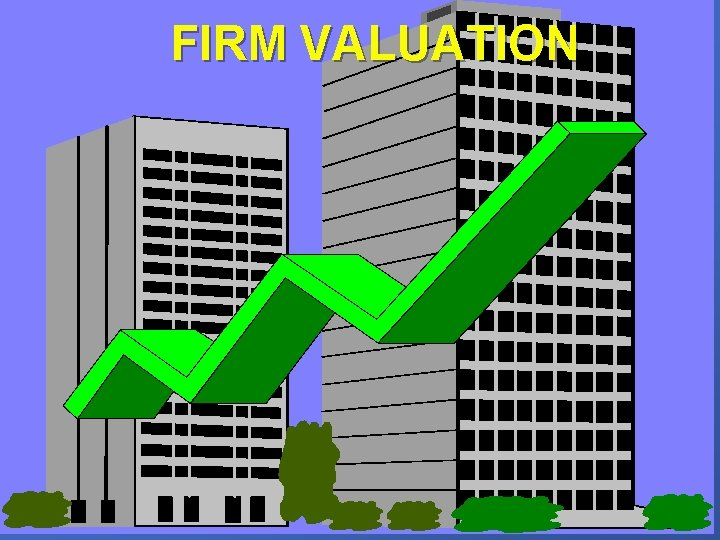FIRM VALUATIONFirm Valuation Assumptions: n Corporate taxes - individual taxe rate is zero n Capital markets are frictionless n Individuals can borrow and lend at the riskfree rate n There are no costs to bankruptcyFirms issue only two types of claims: risk-free debt & (risky) equity n All firms are in the same risk class n No other taxes than corporate taxes n All cash flow streams are perpetuities n Everybody has the same information n No agency costs n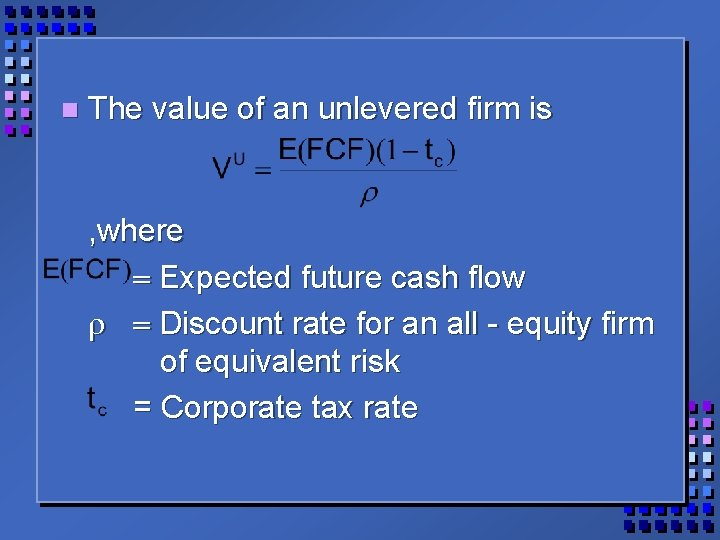n The value of an unlevered firm is , where = Expected future cash flow r = Discount rate for an all - equity firm of equivalent risk = Corporate tax rateIf the firm issues debt, then , where = The amount paid to the lenders, kd = interest rate, D = amount of debt =interest on debt. If the debt is risk-free then.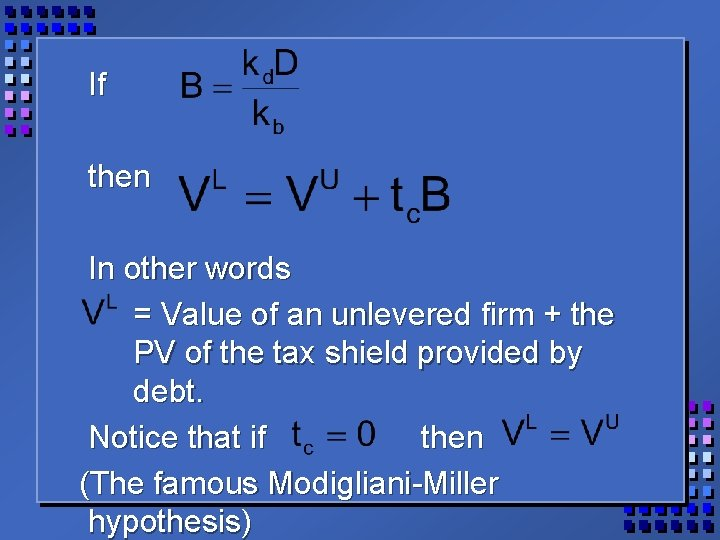If then In other words = Value of an unlevered firm + the PV of the tax shield provided by debt. Notice that if then (The famous Modigliani-Miller hypothesis)This implies that “The market value of any firm is independent of its capital structure and is given by capitalizing its expected return at the rate r appropriate to its risk class” (Modigliani-Miller, American Economic Review, 1958 june)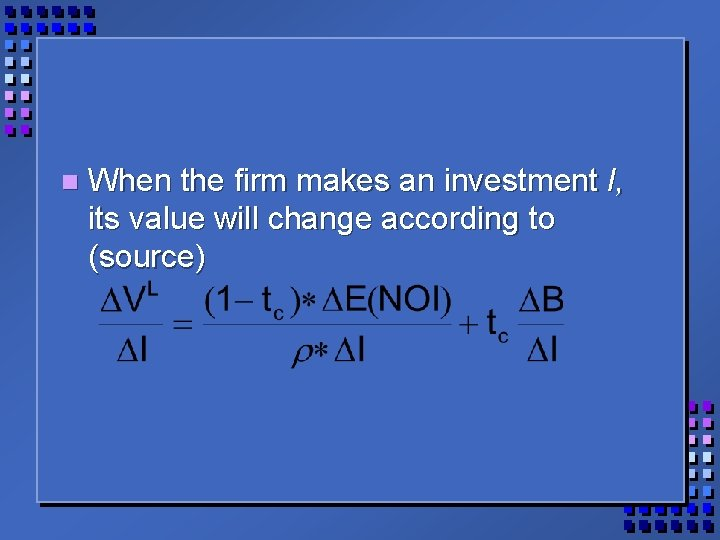n When the firm makes an investment I, its value will change according to (source)n The above investment will affect the value of the levered firm: Note that Equity = old + ds 0+dsn n Because the project has the same risk as those already outstanding, the value of the outstanding debt stays the same.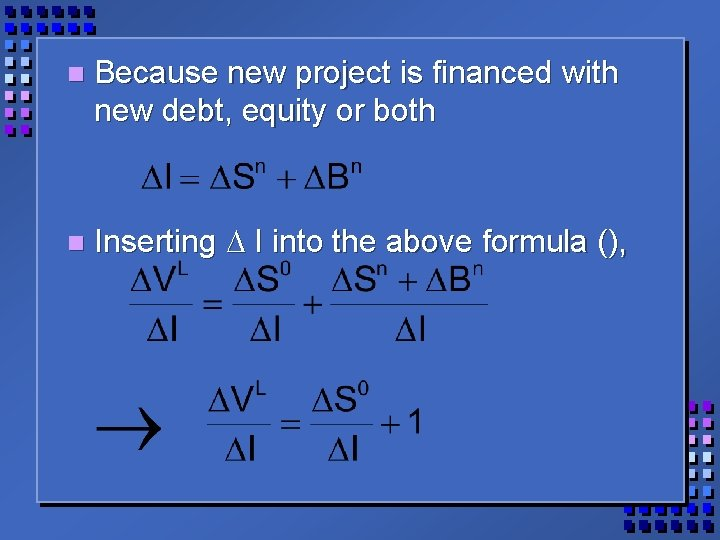n Because new project is financed with new debt, equity or both n Inserting D I into the above formula (),n This means that the project has to increase the shareholders’ wealth, so that and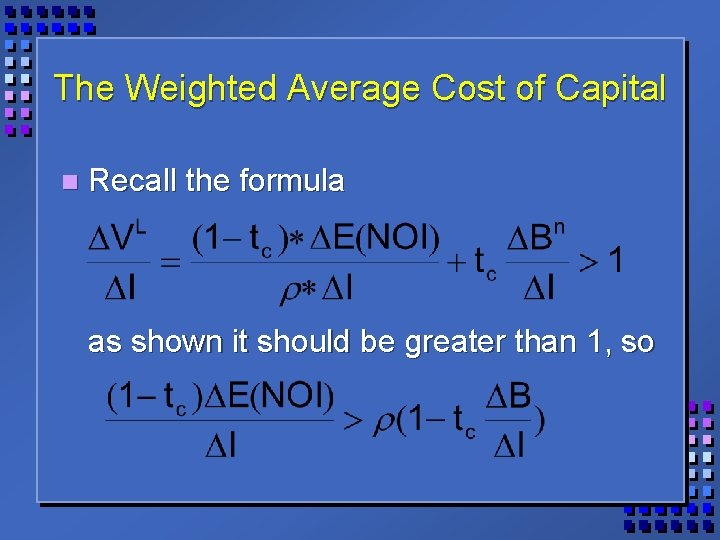The Weighted Average Cost of Capital n Recall the formula as shown it should be greater than 1, son This results in what is called “the Weighted Average Cost of Capital”, WACC, source. n If there are no taxes the cost of capital is independent of capital structure.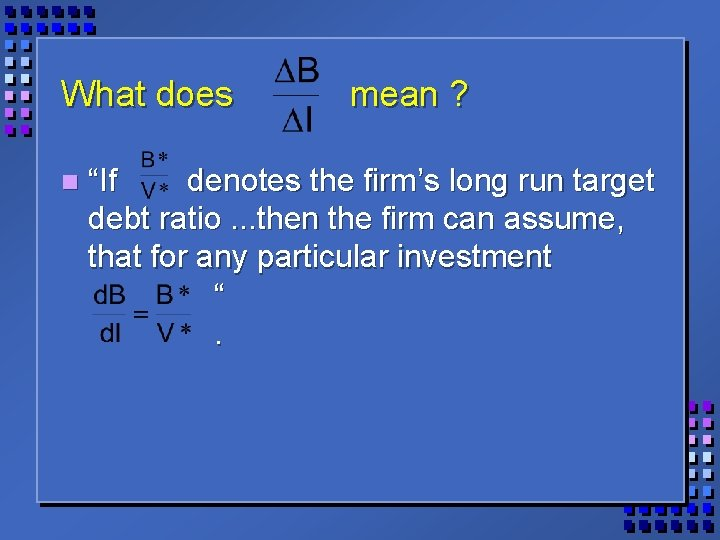What does n mean ? “If denotes the firm’s long run target debt ratio. . . then the firm can assume, that for any particular investment “.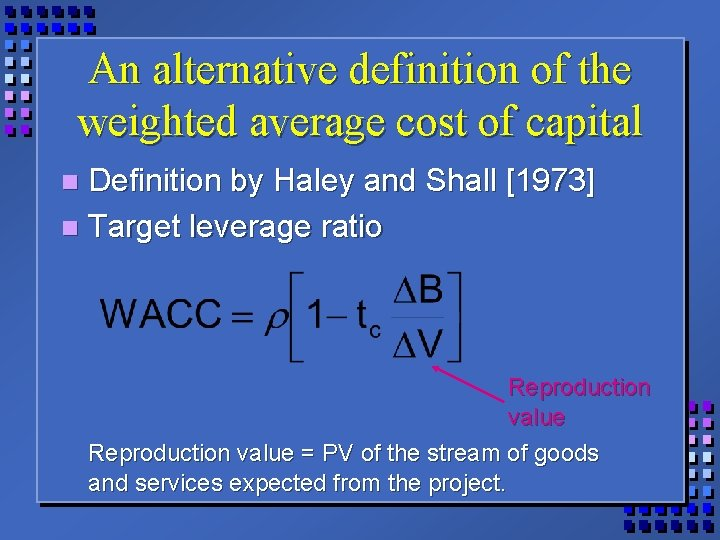An alternative definition of the weighted average cost of capital Definition by Haley and Shall  n Target leverage ratio n Reproduction value = PV of the stream of goods and services expected from the project.How to calculate the cost of the two components in WACC (debt & equity) Assumptions: n The cost of debt = n The cost of equity capital is the return on n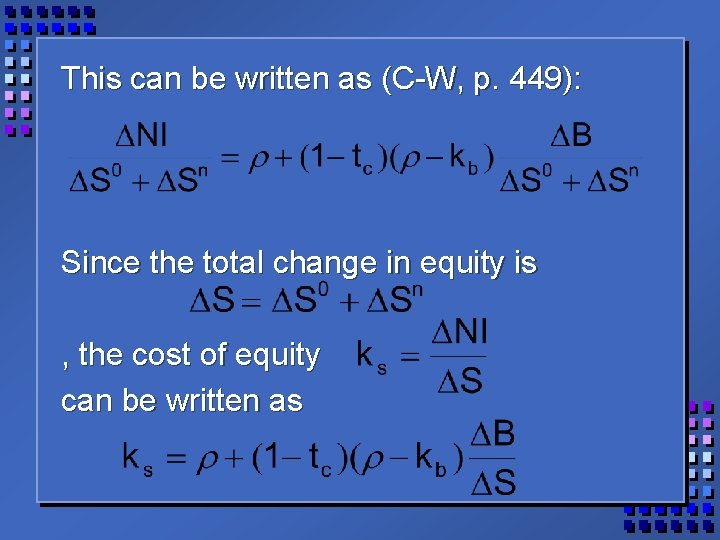This can be written as (C-W, p. 449): Since the total change in equity is , the cost of equity can be written asIf the firm has no debt in its capital structure, then It can be shown that (C-W, 451) WACC can be written as: n tax shield cost of debt Percentage of debt in the cost capital of equity structure Percentage of equity in the capital structure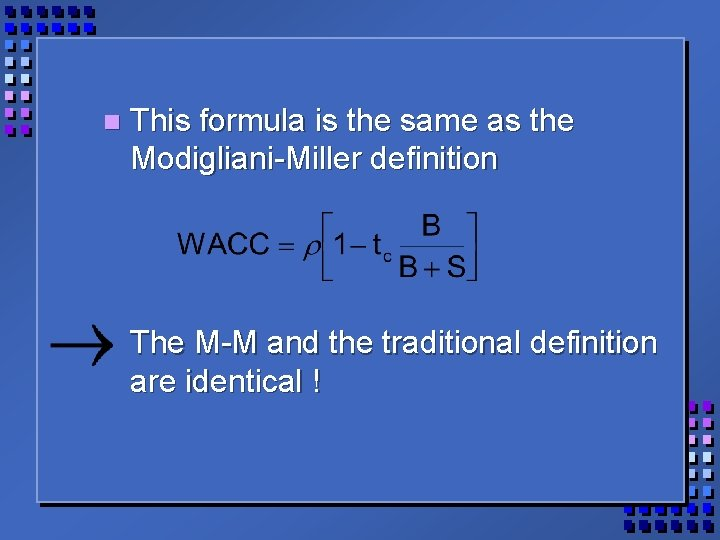n This formula is the same as the Modigliani-Miller definition The M-M and the traditional definition are identical !